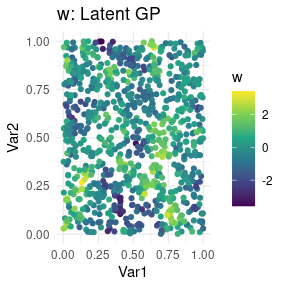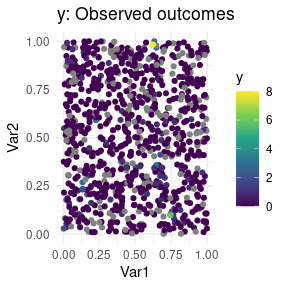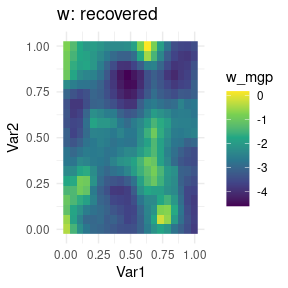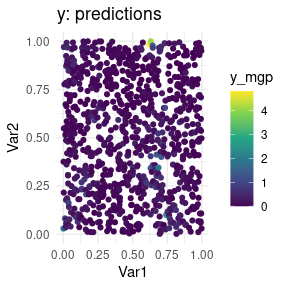# MGPs for univariate non-Gaussian data at irregularly spaced locations

#### 2022-09-19

Compared to the univariate gridded Gaussian case, we now place the data irregularly and assume we observe counts rather than a Gaussian response.

library(magrittr)
library(dplyr)
library(ggplot2)
library(meshed)
set.seed(2021)

SS <- 30 # coord values for jth dimension
dd <- 2 # spatial dimension
n <- SS^2 # number of locations
p <- 3 # number of covariates

# irregularly spaced
coords <- cbind(runif(n), runif(n))
colnames(coords) <- c("Var1", "Var2")

sigmasq <- 1.5
phi <- 10

# covariance at coordinates
CC <- sigmasq * exp(-phi * as.matrix(dist(coords)))
# cholesky of covariance matrix
LC <- t(chol(CC))
# spatial latent effects are a GP
w <- LC %*% rnorm(n)

# covariates and coefficients
X <- matrix(rnorm(n*p), ncol=p)
Beta <- matrix(rnorm(p), ncol=1)

# univariate outcome, fully observed
y_full <- rpois(n, exp(-3 + X %*% Beta + w))

# now introduce some NA values in the outcomes
y <- y_full %>% matrix(ncol=1)
y[sample(1:n, n/5, replace=FALSE), ] <- NA

simdata <- coords %>%
cbind(data.frame(Outcome_full= y_full,
Outcome_obs = y,
w = w))

simdata %>% ggplot(aes(Var1, Var2, color=w)) +
geom_point() +
scale_color_viridis_c() +
theme_minimal() + ggtitle("w: Latent GP")simdata %>% ggplot(aes(Var1, Var2, color=y)) +
geom_point() +
scale_color_viridis_c() +
theme_minimal() + ggtitle("y: Observed outcomes")We now fit the following spatial regression model $y ~ Poisson(\eta),$ where $$log(\eta) = X %*% Beta + w$$, and $$w \sim MGP$$ are spatial random effects. For spatial data, an exponential covariance function is used by default: $$C(h) = \sigma^2 \exp( -\phi h )$$ where $$h$$ is the spatial distance.

The prior for the spatial decay $$\phi$$ is $$U[l,u]$$ and the values of $$l$$ and $$u$$ must be specified. We choose $$l=1$$, $$u=30$$ for this dataset.1

Setting verbose tells spmeshed how many intermediate messages to output while running MCMC. For brevity, we opt to run a very short chain of MCMC with only 2000 iterations, of which we discard the first third. Since the data are irregularly spaced, we build a grid of knots of size 1600 using argument grid_size, which will facilitate computations. Then, just like in the gridded case we use block_size=20 to specify the coarseness of domain partitioning.

Finally, we note that NA values for the outcome are OK since the full dataset is on a grid. spmeshed will figure this out and use settings optimized for partly observed lattices, and automatically predict the outcomes at missing locations. On the other hand, X values are assumed to not be missing.

mcmc_keep <- 200 # too small! this is just a vignette.
mcmc_burn <- 400
mcmc_thin <- 2

mesh_total_time <- system.time({
meshout <- spmeshed(y, X, coords,
family="poisson",
grid_size=c(20, 20),
block_size = 20,
n_samples = mcmc_keep, n_burn = mcmc_burn, n_thin = mcmc_thin,
verbose = 5,
prior=list(phi=c(1,30))
)})
#> Bayesian Meshed GP regression model fit via Markov chain Monte Carlo
#> Sending to MCMC.
#> 20.2% elapsed:    67ms (+   61ms). ETR: 0.29s.
#>   theta: Metrop. acceptance 29.00%, average 35.80%
#>   theta = 2.783 0.175
#>   lambda = 1.893
#>
#> 40.4% elapsed:   124ms (+   57ms). ETR: 0.20s.
#>   theta: Metrop. acceptance 26.50%, average 31.27%
#>   theta = 1.453 0.306
#>   lambda = 2.467
#>
#> 60.5% elapsed:   189ms (+   64ms). ETR: 0.14s.
#>   theta: Metrop. acceptance 21.50%, average 28.51%
#>   theta = 1.283 0.640
#>   lambda = 2.577
#>
#> 80.6% elapsed:   251ms (+   62ms). ETR: 0.07s.
#>   theta: Metrop. acceptance 23.00%, average 26.98%
#>   theta = 1.649 0.748
#>   lambda = 2.196
#>
#> MCMC done [0.303s]

We can now do some postprocessing of the results. We extract posterior marginal summaries for $$\sigma^2$$, $$\phi$$, $$\tau^2$$, and $$\beta_2$$. The model that spmeshed targets is a slight reparametrization of the above:2 $log(\eta) = X \beta + \lambda w,$ where $$w\sim MGP$$ has unitary variance. This model is equivalent to the previous one and in fact we find $$\sigma^2=\lambda^2$$. Naturally, it is much more difficult to estimate parameters when data are counts.

summary(meshout$lambda_mcmc[1,1,]^2) #> Min. 1st Qu. Median Mean 3rd Qu. Max. #> 2.954 4.472 6.076 6.158 7.287 12.585 summary(meshout$theta_mcmc[1,1,])
#>    Min. 1st Qu.  Median    Mean 3rd Qu.    Max.
#>   1.004   1.261   1.561   1.645   1.952   3.718
summary(meshout$beta_mcmc[1,1,]) #> Min. 1st Qu. Median Mean 3rd Qu. Max. #> -0.59923 -0.39025 -0.33478 -0.33508 -0.27247 -0.08278 We proceed to plot predictions across the domain along with the recovered latent effects. We plot the latent effects at the grid we used for fitting spmeshed. Instead, we plot our predictions at the original data locations. We may see some pattern by plotting the data on the log scale. # process means wmesh <- data.frame(w_mgp = meshout$w_mcmc %>% summary_list_mean())
# predictions
ymesh <- data.frame(y_mgp = meshout$yhat_mcmc %>% summary_list_mean()) outdf <- meshout$coordsdata %>%
cbind(wmesh, ymesh) %>%
left_join(simdata, by = c("Var1", "Var2"))

outdf %>% filter(forced_grid==1) %>%
ggplot(aes(Var1, Var2, fill=w_mgp)) +
geom_raster() +
scale_fill_viridis_c() +
theme_minimal() + ggtitle("w: recovered")outdf %>% filter(forced_grid==0) %>%
ggplot(aes(Var1, Var2, color=y_mgp)) +
geom_point() +
scale_color_viridis_c() +
theme_minimal() + ggtitle("y: predictions")1. spmeshed implements a model which can be interpreted as assigning $$\sigma^2$$ a folded-t via parameter expansion if settings$ps=TRUE, or an inverse Gamma with parameters $$a=2$$ and $$b=1$$ if settings$ps=FALSE, which cannot be changed at this time. $$\tau^2$$ is assigned an exponential prior.↩︎

2. At its core, spmeshed implements the spatial factor model $$Y(s) ~ Poisson( exp(X(s)\beta + \Lambda v(s)) )$$ where $$w(s) = \Lambda v(s)$$ is modeled via linear coregionalization.↩︎